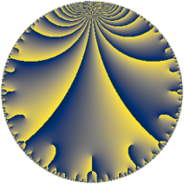# Properties

 Label 43.7.dLevel $43$ Weight $7$ Character orbit 43.d Rep. character $\chi_{43}(7,\cdot)$ Character field $\Q(\zeta_{6})$ Dimension $42$ Newform subspaces $1$ Sturm bound $25$ Trace bound $0$

# Related objects

## Defining parameters

 Level: $$N$$ $$=$$ $$43$$ Weight: $$k$$ $$=$$ $$7$$ Character orbit: $$[\chi]$$ $$=$$ 43.d (of order $$6$$ and degree $$2$$) Character conductor: $$\operatorname{cond}(\chi)$$ $$=$$ $$43$$ Character field: $$\Q(\zeta_{6})$$ Newform subspaces: $$1$$ Sturm bound: $$25$$ Trace bound: $$0$$

## Dimensions

The following table gives the dimensions of various subspaces of $$M_{7}(43, [\chi])$$.

Total New Old
Modular forms 46 46 0
Cusp forms 42 42 0
Eisenstein series 4 4 0

## Trace form

 $$42q - 3q^{3} - 1194q^{4} - 3q^{5} + 111q^{6} - 147q^{7} + 3852q^{9} + O(q^{10})$$ $$42q - 3q^{3} - 1194q^{4} - 3q^{5} + 111q^{6} - 147q^{7} + 3852q^{9} - 981q^{10} - 4104q^{11} + 918q^{12} + 4843q^{13} + 2814q^{14} - 163q^{15} + 36678q^{16} + 3641q^{17} - 507q^{18} - 5229q^{19} - 1599q^{20} - 29990q^{21} + 6575q^{23} - 4622q^{24} + 68484q^{25} + 189q^{26} + 48948q^{28} + 95361q^{29} - 85041q^{30} - 79373q^{31} - 79638q^{33} - 184743q^{34} - 80582q^{35} + 59972q^{36} - 66063q^{37} - 69820q^{38} + 189951q^{40} + 40632q^{41} - 368728q^{43} + 130330q^{44} + 34056q^{46} - 247820q^{47} + 326907q^{48} - 21876q^{49} - 28638q^{50} - 617150q^{52} + 11639q^{53} + 655904q^{54} - 43638q^{55} + 553875q^{56} - 422859q^{57} + 587796q^{58} - 649352q^{59} + 875455q^{60} - 52251q^{61} + 1335249q^{62} + 93690q^{63} - 792420q^{64} + 160395q^{66} - 173603q^{67} + 22286q^{68} + 870417q^{69} + 1670505q^{71} - 2068518q^{72} - 2646201q^{73} - 1625030q^{74} - 80622q^{76} - 486066q^{77} + 2835040q^{78} + 1897639q^{79} - 817059q^{80} + 2215367q^{81} + 1584059q^{83} - 824560q^{84} - 2333682q^{86} - 5547198q^{87} + 2736939q^{89} + 1092196q^{90} - 3724131q^{91} + 470257q^{92} + 488433q^{93} - 1289839q^{95} + 1697650q^{96} + 5260848q^{97} + 4722621q^{98} + 1375834q^{99} + O(q^{100})$$

## Decomposition of $$S_{7}^{\mathrm{new}}(43, [\chi])$$ into newform subspaces

Label Dim. $$A$$ Field CM Traces $q$-expansion
$$a_2$$ $$a_3$$ $$a_5$$ $$a_7$$
43.7.d.a $$42$$ $$9.892$$ None $$0$$ $$-3$$ $$-3$$ $$-147$$